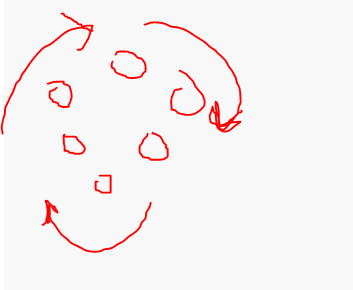# Basic formula request

Someone know how to do a formula Based In math.pi And Tween Service to Make All Parts rotate Smoothly In a Circle Form?

I tried something like this

``````local AmountOfParts = 5

while AmountOfParts, 1 do
local part = Instance.new("Part")
part.Position = amountOfParts + math.pi/part.Position
end
``````

I’m confused by what you’re trying to do, are you trying to make a circle with parts? or make a part orbit another part?Rotate around

Im assuming this is what you wanted, with some basic polar cordinates

no

charchar

Is this what you wanted?

Yes, why use math.pi instead of writing 3?

3 is equals to rougly 171.887 degrees. but with the precision of math.pi, we can get to rougly 180 degrees.

1 Like